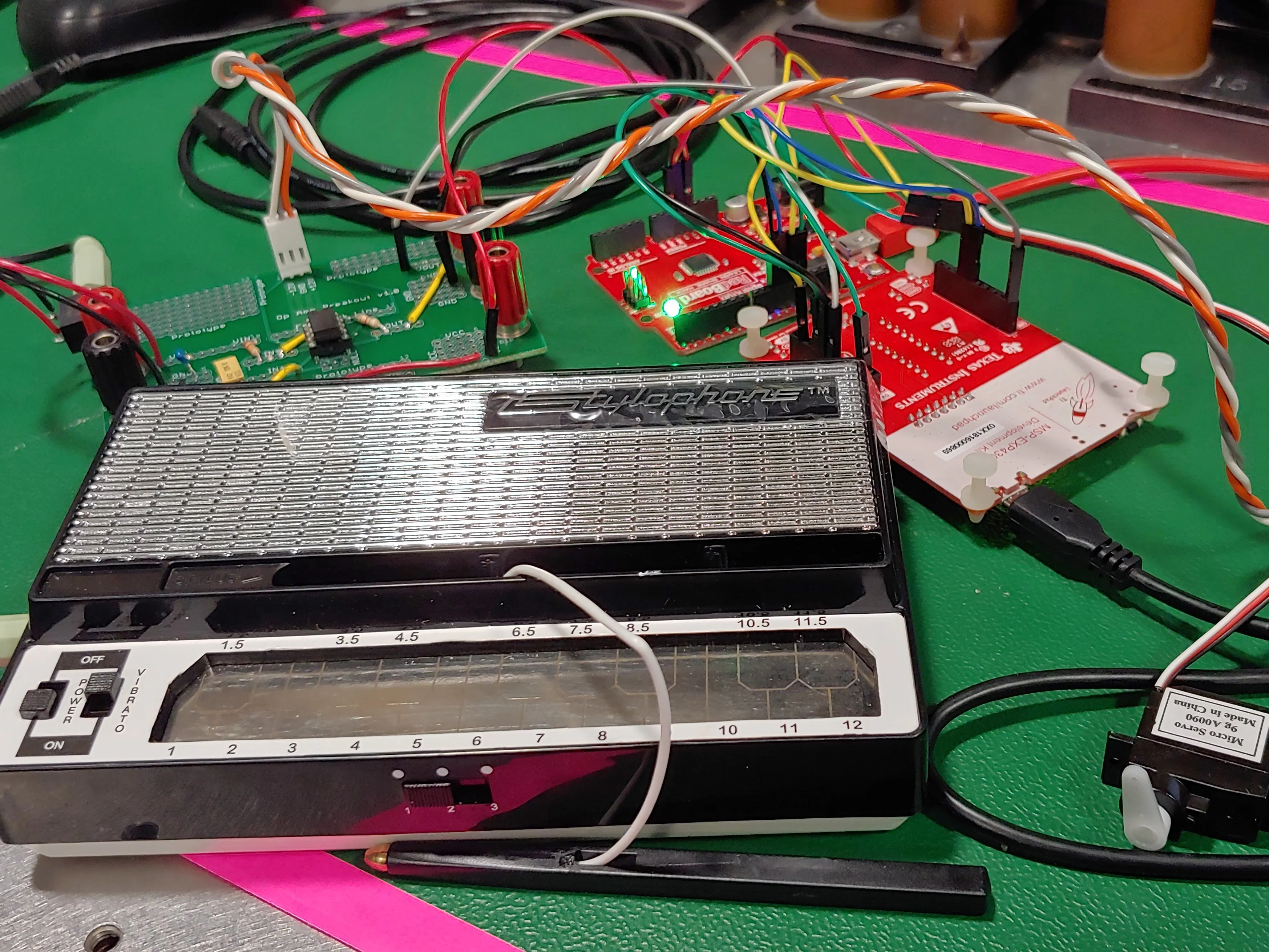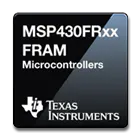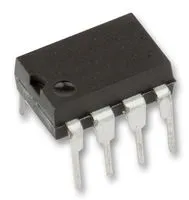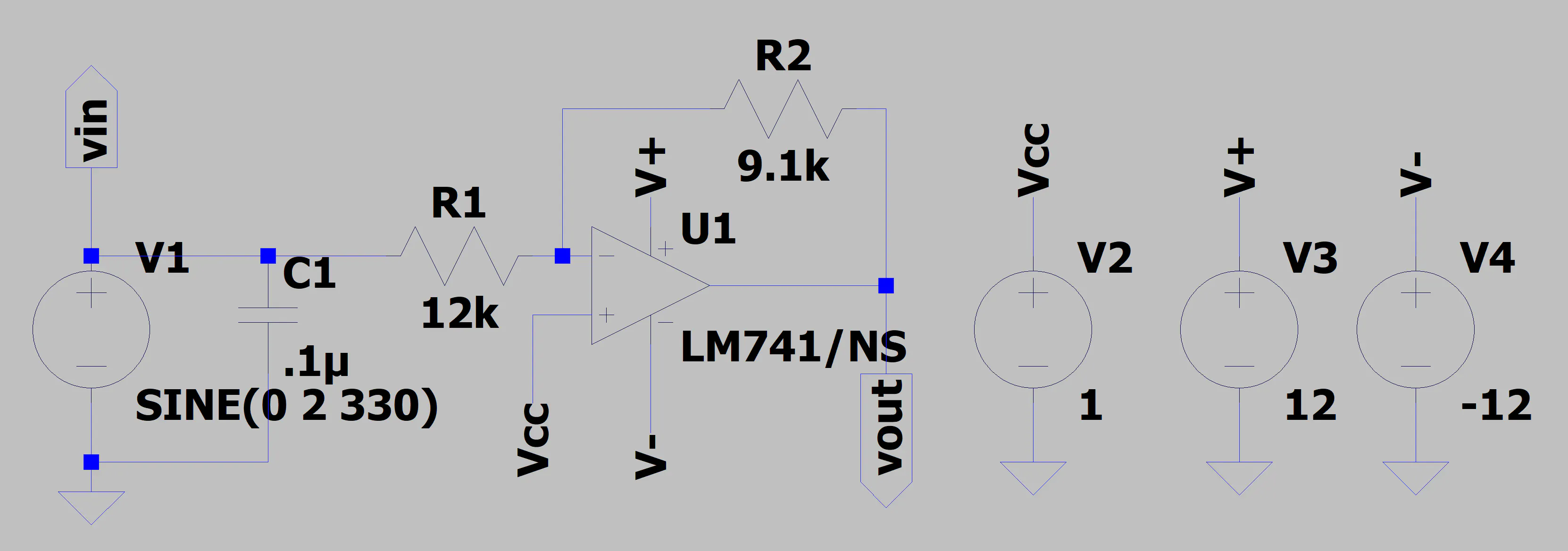# Controlling Servos with a Stylophone: Pitch Detection

The stylophone was one of the wackiest forgotten toys of the 1960s and '70s, and today, "we have the technology" to make it better.

IntermediateFull instructions provided1,228## Things used in this project

### Hardware componentsTexas Instruments MSP430 Microcontroller TI MSP430G2553
×1Arduino UNO Redboard
×1Texas Instruments General Purpose Dual Op-Amp TI LM741
×1
 Servo Module (Generic) Micro-servo
×1

### Software apps and online servicesTexas Instruments Code Composer Studio CCS 8.1.0Arduino IDE

### Hand tools and fabrication machinesHot glue gun (generic)Soldering iron (generic)

## Schematics

### Gain and shift circuit

requires the LM741 SPICE model to work

### Gain and shift circuit schematic## Code

### MSP430G2553 Master Code

C/C++
```/******************************************************************************
MSP430G2553 Project Creator

SE 423  - Dan Block
Spring(2019)

Written(by) : Steve(Keres)
College of Engineering Control Systems Lab
University of Illinois at Urbana-Champaign
*******************************************************************************/

#include "msp430g2553.h"
#include "UART.h"

void print_every(int rate);

char newprint = 0;
int timecheck = 0;
long millivolts = 0;
int statevar = 0;
unsigned char MISO_val = 0;

void main(void) {

WDTCTL = WDTPW + WDTHOLD;                 // Stop WDT

if (CALBC1_16MHZ ==0xFF || CALDCO_16MHZ == 0xFF) while(1);

DCOCTL = CALDCO_16MHZ;    // Set uC to run at approximately 16 Mhz
BCSCTL1 = CALBC1_16MHZ;

// Control Register 0
ADC10CTL0 = SREF_0; // Vr+ = Vcc and Vr- = Vss (default)
// REFOUT: reference output off (default)
// REFBURST: continuous (default)
// MSC: single conversion mode (default)
// REFON: reference generator off (default)
// Control Register 1
ADC10CTL1 = INCH_3; //input channel A3 (default)
// ADC10DF: straight binary data format (default)
ADC10CTL1 += CONSEQ_0; // Single channel single conversion
// analog enable control register 0

// Init Port 2
// setup P2.1 with Timer1_A3.TA1 and P2.4 with Timer1_A3.TA2
P2DIR |= 0x12;
P2SEL |= 0x12;
P2SEL2 = 0;

// Initialize Port 1, P1.0 LED, P1.4 SS
P1SEL &= ~(BIT0 + BIT4);                  // P1.0 and P1.4 GPIO
P1SEL &= ~(BIT0 + BIT4);                  // P1.0 and P1.4 GPIO
P1REN &= ~(BIT0 + BIT4);                  // P1.0 and P1.4 Resistor disabled
P1DIR |= BIT0 + BIT4;                     // Set P1.0 and P1.4 to output direction
P1OUT &= BIT4;  // Initially set P1.4/SS high

// Port 1 SPI pins, P1.5 SCLK, P1.6 MISO, P1.7 MOSI
P1SEL |= BIT5 + BIT7 + BIT6;              // Secondary Peripheral Module Function for P1.5-1.7
P1SEL2 |= BIT5 + BIT7 + BIT6;             // Secondary Peripheral Module Function for P1.5-1.7

// Polarity and SCLK for SPI
UCB0CTL0 = UCCKPH + UCCKPL;    // first edge: data capture, following edge: data update , SCLK inactive state High

// Initialize SPI
UCB0CTL0 |= UCMSB + UCMST + UCSYNC + UCMODE_0;  // MSB first, master, 3-pin, 8-bit synchronous
UCB0CTL1 = UCSSEL_2 + UCSWRST;               // SMCLK, enable SW Reset
// bit rate: SMCLK/x=SCLK
// smclk same as arduino uno (16 Mhz)
UCB0BR0 = 32;                                 // low byte, divide by
// 4 divider or greater, 8 and 16 causes issues, 32 seems okay
UCB0BR1 = 0;                                 // same as 1 divider, high byte
UCB0CTL1 &= ~UCSWRST;                        // **Initialize USCI state machine**
IFG2 &= ~UCB0RXIE;                        // Clear RX interrupt flag in case it was set during init
IE2 |= UCB0RXIE;                          // Enable USCI0 RX interrupt

// Timer A Config
// use Timer0_A3 to init 1ms timed interrupt
TACCTL0 = CCIE;             // Enable Periodic interrupt
TACCR0 = 2000;                // period = 1ms
TACTL = TASSEL_2 + MC_1; // source SMCLK, up mode

// change TA1CCR0 counting rate since it is a 16 bit register
TA1CCR0 = 40000;                             // PWM Freq = 50Hz, 1/(50 Hz/16MHz)/8 = 655
TA1CCTL0 = 0;
TA1CCTL1 = OUTMOD_7;                         // TA1CCR1 reset/set
TA1CCR1 = 2000;                               // TA1CCR1 PWM duty cycle, TA1CCR0/TA1CCR1
TA1CCTL2 = OUTMOD_7;
TA1CCR2 = 2000;                           // 0 deg: (0.6ms/20ms)*40000
TA1CTL = TASSEL_2 + MC_1 + ID_3;                  // source SMCLK, up mode, divide 8

Init_UART(115200,1);    // Initialize UART for 115200 baud serial communication

_BIS_SR(GIE);       // Enable global interrupt

while(1) {  // Low priority Slow computation items go inside this while loop.  Very few (if anyt) items in the HWs will go inside this while loop

// for use if you want to use a method of receiving a string of chars over the UART see USCI0RX_ISR below
//      if(newmsg) {
//          newmsg = 0;
//      }

// The newprint variable is set to 1 inside the function "print_every(rate)" at the given rate
if ( (newprint == 1) && (senddone == 1) )  { // senddone is set to 1 after UART transmission is complete

// only one UART_printf can be called every 15ms
UART_printf("mV:%ld MISO:%d\n\r",millivolts,MISO_val);

newprint = 0;
}

}
}

// Timer A0 interrupt service routine
#pragma vector=TIMER0_A0_VECTOR
__interrupt void Timer_A (void)
{
timecheck++; // Keep track of time for main while loop.
print_every(5000);  // units determined by the rate Timer_A ISR is called, print every "rate" calls to this function
// make sure to not print too long of a string, stops working
switch(MISO_val){
case 10: //A
TA1CCR2 = 2000;     // 0 deg: (0.6ms/20ms)*40k
TA1CCR1 = 2000;
break;
case 15:
TA1CCR2 = 2100;
TA1CCR1 = 2100;
break;
case 20: //B
TA1CCR2 = 2200;
TA1CCR1 = 2200;
break;
case 30: //C
TA1CCR2 = 2400;
TA1CCR1 = 2400;
break;
case 35:
TA1CCR2 = 2500;
TA1CCR1 = 2500;
break;
case 40: //D
TA1CCR2 = 2600;
TA1CCR1 = 2600;
break;
case 45:
TA1CCR2 = 2700;
TA1CCR1 = 2700;
break;
case 50: //E
TA1CCR2 = 2800;
TA1CCR1 = 2800;
break;
case 60: //F
TA1CCR2 = 3000;
TA1CCR1 = 3000;
break;
case 65:
TA1CCR2 = 3100;
TA1CCR1 = 3100;
break;
case 70: //G
TA1CCR2 = 3200;
TA1CCR1 = 3200;
break;
case 75:
TA1CCR2 = 3300;
TA1CCR1 = 3300;
break;
case 80: //A
TA1CCR2 = 3400;
TA1CCR1 = 3400;
break;
case 85:
TA1CCR2 = 3500;
TA1CCR1 = 3500;
break;
case 90: //B
TA1CCR2 = 3600;
TA1CCR1 = 3600;
break;
case 100: //C
TA1CCR2 = 3800;
TA1CCR1 = 3800;
break;
case 105:
TA1CCR2 = 3900;
TA1CCR1 = 3900;
break;
case 110: //D
TA1CCR2 = 4000;     // 180 deg: (3ms/20ms)*40k
TA1CCR1 = 4000;
break;
case 115:
TA1CCR2 = 4100;
TA1CCR1 = 4100;
break;
case 120: //E
TA1CCR2 = 4200;
TA1CCR1 = 4200;
break;
default:
break;
}
}

// ADC 10 ISR - Called when a sequence of conversions (A7-A0) have completed

P1OUT &= ~0x10; // pull SS (P1.4) low
UCB0TXBUF = (adc_raw >> 8); // send MSByte

}

// USCI Transmit ISR - Called when TXBUF is empty (ready to accept another character)
#pragma vector=USCIAB0TX_VECTOR
__interrupt void USCI0TX_ISR(void) {

if(IFG2&UCA0TXIFG) {        // USCI_A0 requested TX interrupt
if(printf_flag) {
if (currentindex == txcount) {
senddone = 1;
printf_flag = 0;
IFG2 &= ~UCA0TXIFG;
} else {
UCA0TXBUF = printbuff[currentindex];
currentindex++;
}
} else if(UART_flag) {
if(!donesending) {
UCA0TXBUF = txbuff[txindex];
if(txbuff[txindex] == 255) {
donesending = 1;
txindex = 0;
}
else txindex++;
}
} else {  // interrupt after sendchar call so just set senddone flag since only one char is sent
senddone = 1;
}

IFG2 &= ~UCA0TXIFG;
}

if(IFG2&UCB0TXIFG) {    // USCI_B0 requested TX interrupt (UCB0TXBUF is empty)

IFG2 &= ~UCB0TXIFG;   // clear IFG
}
}

// USCI Receive ISR - Called when shift register has been transferred to RXBUF
// Indicates completion of TX/RX operation
#pragma vector=USCIAB0RX_VECTOR
__interrupt void USCI0RX_ISR(void) {

if(IFG2&UCB0RXIFG) {  // USCI_B0 requested RX interrupt (UCB0RXBUF is full)
P1OUT |= 0x10; // pull SS (P1.4) high
receive_lsb = 1; // next byte is lsbyte
MISO_val = (UCB0RXBUF & 0xFF);
P1OUT &= ~0x10; // pull SS (P1.4) low
UCB0TXBUF = adc_raw & 0xFF; // send LSByte
}
else {
P1OUT |= 0x10; // pull SS (P1.4) high
receive_lsb = 0; // next byte is msbyte
MISO_val = (UCB0RXBUF & 0xFF);
}
IFG2 &= ~UCB0RXIFG;   // clear IFG
}

if(IFG2&UCA0RXIFG) {  // USCI_A0 requested RX interrupt (UCA0RXBUF is full)

//    Uncomment this block of code if you would like to use this COM protocol that uses 253 as STARTCHAR and 255 as STOPCHAR
/*      if(!started) {  // Haven't started a message yet
if(UCA0RXBUF == 253) {
started = 1;
newmsg = 0;
}
}
else {  // In process of receiving a message
if((UCA0RXBUF != 255) && (msgindex < (MAX_NUM_FLOATS*5))) {
rxbuff[msgindex] = UCA0RXBUF;

msgindex++;
} else {    // Stop char received or too much data received
if(UCA0RXBUF == 255) {  // Message completed
newmsg = 1;
rxbuff[msgindex] = 255; // "Null"-terminate the array
}
started = 0;
msgindex = 0;
}
}
*/

IFG2 &= ~UCA0RXIFG;
}

}

// This function takes care of all the timing for printing to UART
// Rate determined by how often the function is called in Timer ISR
int print_timecheck = 0;
void print_every(int rate) {
if (rate < 15) {
rate = 15;
}
if (rate > 10000) {
rate = 10000;
}
print_timecheck++;
if (print_timecheck == rate) {
print_timecheck = 0;
newprint = 1;
}

}
```

### Arduino Uno Slave Code

Arduino
```/*
* SPI Slave, Arduino Uno
* 10 (SS), 11 (MOSI), 12 (MISO), 13 (SCK)
*/
#include "pins_arduino.h"

/*
*  SPI global vars
*  modified code from
*/
volatile byte pos;
volatile boolean process_it;
long loop_count = 0;
byte MOSI_val = 0;
unsigned long millivolts = 0;
unsigned char note = 1; //max val of 127, wraps back around

// variables for frequency detection
float frequency = 0.0;
int clocks = 0;
int clocks_arr[] = {0,0,0,0,0,0,0,0,0,0};
int clocks_arr_sorted[] = {0,0,0,0,0,0,0,0,0,0};
int clocks_arr_size = 10;
unsigned int samps[] = {0,0,0,0};
int samps_arr_size = 4;

void setup (void) {
Serial.begin (9600);   // debugging

// have to send on master in, *slave out*
pinMode(MISO, OUTPUT);

// turn on SPI with interrupts, slave mode, msbit first
// clock idle when high, sample on falling edge of clock (see MSP430x2xx user guide pg448, 451)
SPCR = _BV(SPE) + _BV(SPIE) + _BV(CPOL) +_BV(CPHA);

/*
*  SPIE - Enables the SPI interrupt when 1
*  SPE - Enables the SPI when 1
*  DORD - Sends data least Significant Bit First when 1, most Significant Bit first when 0
*  MSTR - Sets the Arduino in master mode when 1, slave mode when 0
*  CPOL - Sets the data clock to be idle when high if set to 1, idle when low if set to 0
*  CPHA - Samples data on the falling edge of the data clock when 1, rising edge when 0
*  SPR1 and SPR0 - Sets the SPI speed, 00 is fastest (4MHz) 11 is slowest (250KHz)
*
*/

pos = 0;
process_it = false;
}  // end of setup

void quickSort(int arr[], int left, int right) {
int i = left, j = right;
int tmp;
int pivot = arr[(left + right) / 2];

/* partition */
while (i <= j) {
while (arr[i] < pivot)
i++;
while (arr[j] > pivot)
j--;
if (i <= j) {
tmp = arr[i];
arr[i] = arr[j];
arr[j] = tmp;
i++;
j--;
}
};

/* recursion */
if (left < j)
quickSort(arr, left, j);
if (i < right)
quickSort(arr, i, right);
}

void newSample(){
// stores last few adc raw values
int i = 0;
for (i=0; i<samps_arr_size; i++){
if (i == samps_arr_size-1) samps[i] = adc_raw;
else samps[i] = samps[i+1];
}

//calculates average
for (i=0; i<samps_arr_size; i++){
}

/*
*  since input is like sine wave, one peak per period
*  trigger on rise at 700 (~2V), near the top of the wave
*/
if (clocks > 10 && clocks < 80){
// keep last few clock values
for (i=0; i<clocks_arr_size; i++){
if (i == clocks_arr_size-1) clocks_arr[i] = clocks;
else clocks_arr[i] = clocks_arr[i+1];
}
for (i=0; i<clocks_arr_size; i++){
clocks_arr_sorted[i] = clocks_arr[i];
}
// sort values
quickSort(clocks_arr_sorted,0,clocks_arr_size-1);
// get median value
clocks = clocks_arr_sorted[(clocks_arr_size+1)/2];
// find frequency using curve of best fit
if (clocks >= 38){
frequency = (float)((long)clocks*(long)clocks*(long)clocks)*-0.0023 + (float)((long)clocks*(long)clocks)*0.4059 - 26.337*(float)clocks + 735.56;
}
else if (clocks >= 36) frequency = 207.65;
else if (clocks >= 34) frequency = 220.0;
else if (clocks >= 32) frequency = 233.08;
else if (clocks >= 30) frequency = 246.94;
else if (clocks >= 28) frequency = 261.63;
else if (clocks >= 26) frequency = 277.18;
else if (clocks >= 25) frequency = 293.66;
else if (clocks >= 23) frequency = 311.13;
else if (clocks >= 22) frequency = 329.63;
else frequency = 330.;

}
clocks = 0;
}
else clocks++;

}

// SPI interrupt routine
ISR (SPI_STC_vect) {
MOSI_val = SPDR;
SPDR = note*2; // send back char to MSP430
// transferred value is haved for some reason
}
else {
process_it = true;
}

}

void noteDetect(){
if(frequency > 0){
if (frequency < 113) note = 10;         //A 110 + 3.0 Hz
else if (frequency < 119.54) note = 15; //  116.54 + 3.0 Hz
else if (frequency < 126.47) note = 20; //B 123.47 + 3.0 Hz
else if (frequency < 134.81) note = 30; //C 130.81 + 4.0 Hz
else if (frequency < 142.59) note = 35; //  138.59 + 4.0 Hz
else if (frequency < 150.83) note = 40; //D 146.83 + 4.0 Hz
else if (frequency < 159.56) note = 45; //  155.56 + 4.0 Hz
else if (frequency < 169.81) note = 50; //E 164.81 + 5.0 Hz
else if (frequency < 179.61) note = 60; //F 174.61 + 5.0 Hz
else if (frequency < 190) note = 65;    //  185 + 5.0 Hz
else if (frequency < 201) note = 70;    //G 196 + 5.0 Hz
else if (frequency < 212.65) note = 75; //  207.65 + 5.0 Hz
else if (frequency < 226) note = 80;    //A 220 + 6.0 Hz
else if (frequency < 239.08) note = 85; //  233.08 + 6.0 Hz
else if (frequency < 253.94) note = 90; //B 246.94 + 7.0 Hz
else if (frequency < 268.63) note = 100; //C 261.63 + 7.0 Hz
else if (frequency < 285.18) note = 105; // 277.18 + 8.0 Hz
else if (frequency < 302.66) note = 110; //D 293.66 + 9.0 Hz
else if (frequency < 320.13) note = 115; // 311.13 + 9.0 Hz
else if (frequency < 338.63) note = 120; //E 329.63 + 9.0 Hz
else {
// debugging
if (note == 10) note = 120;
//else note = 10;
}
}
}

// main loop - wait for flag set in interrupt routine
void loop (void) {
loop_count++;

if (process_it) {
//Serial.print("mV: ");
//Serial.println(millivolts);
pos = 0;
process_it = false;
newSample();
noteDetect();
if  (loop_count%1000 == 0){
//Serial.print(millivolts);
//Serial.print(" mv ");
Serial.print(frequency);
Serial.println(" hz ");
}
}  // end of flag set

}  // end of loop
```

## Credits

### Keith

2 projects • 1 follower